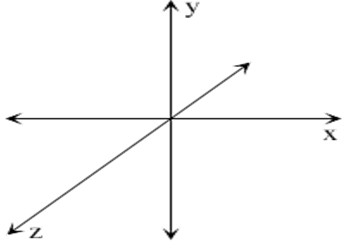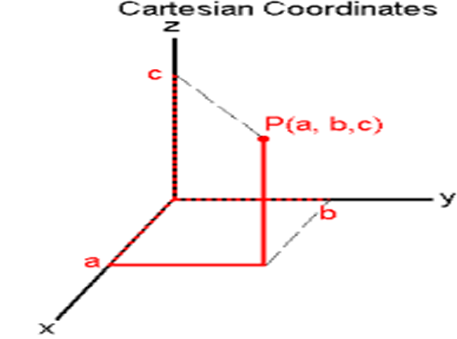#### The equation of x-axis in space are Option 1)Option 2)Option 3)Option 4)As we learnt in the concept

Co-ordinate axes -

There are infinite points in plane we want to identify them by using three mutually perpendicular lines known as co-ordinate axes- whereinCo-ordinate of a point -

Consider a point P(a,b,c) in space and drop a perpendicular from it on XY plane the algebraic length of this perpendicular is called z cordinate. Now from this point draw perpendiculars to X and Y axes, the algebraic length of these perpendiculars is known as x and y co-ordinate respectively.

- whereiny=0, Z=0

Option 1)Option is incorrect

Option 2)Option is incorrect

Option 3)Option is incorrect

Option 4)Option is correct